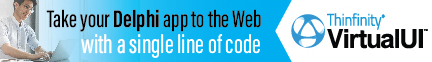DelphiBasicsDefaultDirective Defines default processing for a property
 1 Indexed property declaration; Default 2 Ordinal property declaration Default Ordinal constant;
Description
The Default directive is used in two separate, and quite different ways with properties to establish default processing.

Version 1

When you have one or more properties that used an index as a parameter (see the example and Index for further details), you can specify the Default directive. It allows you to use a more compact way of using the property.

Instead of :

myValue := MyObject.GetItem(23);

We can use :

myValue := MyObject;

This can make for more readable and compact code, but can be confusing for newcomers to Delphi - they will see MyObject is not an array and find it difficult to find the mechanism. Especially if the class is big.

Version 2

Has a much more specialised use. It stores the supplied default ordinal property value in the run time information for the class. Its use is beyond the scope of Delphi Basics.
Related commands
 Private Starts the section of private data and methods in a class Property Defines controlled access to class fields Protected Starts a section of class private data accesible to sub-classes Public Starts an externally accessible section of a class Published Starts a published externally accessible section of a class
Author linksDownload this web site as a Windows program.

 Example code : A simple example // Full Unit code.// -----------------------------------------------------------// You must store this code in a unit called Unit1 with a form// called Form1 that has an OnCreate event called FormCreate. unit Unit1; interface uses   Windows, Messages, SysUtils, Variants, Classes, Graphics, Controls, Forms,   Dialogs; type  // Class with Indexed properties   TRectangle = class   private     fCoords: array[0..3] of Longint;     function  GetCoord(Index: Integer): Longint;     procedure SetCoord(Index: Integer; Value: Longint);   public     property Left   : Longint Index 0 read GetCoord write SetCoord;     property Top    : Longint Index 1 read GetCoord write SetCoord;     property Right  : Longint Index 2 read GetCoord write SetCoord;     property Bottom : Longint Index 3 read GetCoord write SetCoord;     property Coords[Index: Integer] : Longint              read GetCoord write SetCoord; Default;   end;   // The form class itself   TForm1 = class(TForm)     procedure FormCreate(Sender: TObject);   end; var   Form1: TForm1; implementation {\$R *.dfm} // TRectangle property 'Getter' routine function TRectangle.GetCoord(Index: Integer): Longint; begin  // Only allow valid index values   if (Index >= 0) and (Index <= 3)   then Result := fCoords[Index]   else Result := -1; end; // TRectangle property 'Setter' routine procedure TRectangle.SetCoord(Index, Value: Integer); begin  // Only allow valid index values   if (Index >= 0) and (Index <= 3)   then fCoords[Index] := Value; end; // Main line code procedure TForm1.FormCreate(Sender: TObject); var   myRect : TRectangle; begin  // Create my little rectangle   myRect := TRectangle.Create;   // And set the corner coordinates  myRect.Left := 22;       // Left using direct method   myRect.Top  := 33;  myRect.SetCoord(2,44);   // Right using indexed method   myRect.SetCoord(3,55);   // Get the first two coords using the get method name   ShowMessage('myRect coord 0 = '+intToStr(myRect.GetCoord(0)));   ShowMessage('myRect coord 0 = '+intToStr(myRect.GetCoord(1)));   // Now use the more compact version to get the remaining coords  // This is only possible when we have a 'default' indexed property   ShowMessage('myRect coord 1 = '+intToStr(myRect));   ShowMessage('myRect coord 1 = '+intToStr(myRect)); end; end. myRect coord 0 = 22   myRect coord 1 = 33   myRect coord 2 = 44   myRect coord 3 = 55
 Delphi Programming © Neil Moffatt 2002 - 2020. All rights reserved.  |  Home Page